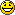# Why is the wave function a complex number##Why is the wave function a complex number

Basically the title tells u exactly what my question is, Why do we take psi i.e; the wave function as a complex quantity

##Re: Why is the wave function a complex number

The question that lonestar raised is a very important one since in most of the literature it is just suggested as if it was a postulate that scodinger got from thin air and forgets to mention the series of events and thought experiments involved. One of the leading scintists (I forgot his name) said that even physics donot bother about its origins; but that is not how science works.

So here I am trying to go through the thoughts of scodinger which led to his postulate. Some of the observation's are from the book QUANTUM MECHANICS by JAMES & BARTLETT . So I would like you to read that book to understand the idea better and then to criticise it.

In the year 1924 De Broglie proposed his postulate which lead to the idea of matter waves and the prediction of matter waves naturally lead to discussions on what the amplitude of matter waves was. Scodinger might have first considered a function of the form Y=ASin(kx-wt), where A is a real constant. But this function although is perfectly in agreement (mathematicaly) with the general (classical) wave equation d^2Y/dt^2= v^2×d^2Y/dx^2.......(1) .There was a major problem. According to the concept of a matter wave the velocity of the wave should be equal to the velocity of the particle as the particle was itself the wave (I WOULD LIKE TO STRESS THE FACT THAT THIS WAS WRONG AS LATER SHOWN,But as we know scodinger himself was not clear of what it was so we cannot blame him to think that way.) So he might have disided to come up with some other general equation.

For this he might have played around with the Hamiltonian (refer the book)and might have come up with the time independent scodinger equation. This is satisfied by equation (1). Till now there is no need of complex constants. But the real fun starts when he tries to come up with a time dependent equation.

When he tries to do it on time (read the book) the equation so formed is actually a non leniar one and we know that in classical waves it is always leniar so he wanted to create a leniar equation.

He might have desperately tried out the function Y=e^(i (kx-wt)). This function satisfies the time independent scodinger equation at the same time provides a linear equation for the time depended form. The only problem was that it involved complex parts. But upon trying it out on hydrogen atom the equation predicted everything accurately. So he published the equation as a postulate in 1926. The mathematical manipulations used and interpretations are not refined and as result it can only be considered to be pseudo derivation. Not a derivation.

If there is any objections pleas do oppose.

##Re: Why is the wave function a complex number

The problem with the above discussion is that it is speculative at best. Nobody knows what Schrodinger thought apart from the speculations. As I mentioned in the class that the equation without mass (EMW) and with mass take different forms. With mass, the imaginary part naturally arises to provide real eigenvalues (observables). The "derivation" from wave equation to Schrodinger equation is also proposed as provided in the linked manuscript. Note that it is not a peer reviewed publication, though. Therefore, it cannot stand as However, it is not the distinctive proof that Schrodinger came up with this. There are other approaches as well. Therefore, science does work this way that when we don't know the origin, we throw in postulates.
This is most probable logical steps.

##Re: Why is the wave function a complex number

Read the class notes properly and there you see it at a corner......CLEARLY WRITTEN!!!!##Re: Why is the wave function a complex number